# Instant Centers of Velocities Introduction Instant Centers of

• Slides: 15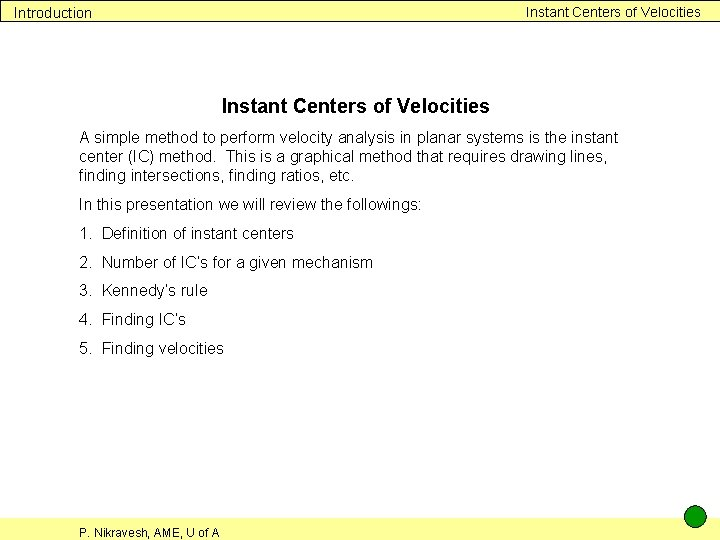Instant Centers of Velocities Introduction Instant Centers of Velocities A simple method to perform velocity analysis in planar systems is the instant center (IC) method. This is a graphical method that requires drawing lines, finding intersections, finding ratios, etc. In this presentation we will review the followings: 1. Definition of instant centers 2. Number of IC’s for a given mechanism 3. Kennedy’s rule 4. Finding IC’s 5. Finding velocities P. Nikravesh, AME, U of A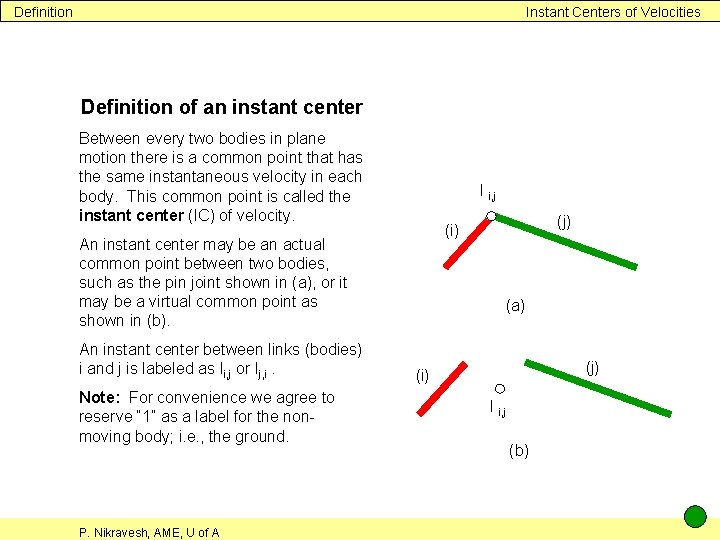Definition Instant Centers of Velocities Definition of an instant center Between every two bodies in plane motion there is a common point that has the same instantaneous velocity in each body. This common point is called the instant center (IC) of velocity. I i, j An instant center may be an actual common point between two bodies, such as the pin joint shown in (a), or it may be a virtual common point as shown in (b). An instant center between links (bodies) i and j is labeled as Ii, j or Ij, i. Note: For convenience we agree to reserve “ 1” as a label for the nonmoving body; i. e. , the ground. P. Nikravesh, AME, U of A (j) (i) (a) (j) (i) I i, j (b)Obvious instant centers Instant Centers of Velocities Obvious instant centers Some instant centers are very easy to identify: 1. Two bodies connected by a pin joint: The center of the pin joint is the IC between the two bodies as shown in (a). Since the pin joint remains between the bodies as they move, this IC is a permanent center as well. 2. Two bodies connected by a sliding joint: Since the two bodies rotate together; i. e. , there is no relative rotation between them, the instant center is in infinity on an axis perpendicular to the axis of sliding as shown in (b). Note: ωi = ωj P. Nikravesh, AME, U of A I i, j (j) (i) (a) I i, j (j) (i) (b)Number of instant centers Instant Centers of Velocities Number of instant centers For a mechanism containing n links, there are n (n − 1) ∕ 2 instant centers. For example for a fourbar mechanism, there are 4 (4 − 1) ∕ 2 = 6 instant centers. The same is true for or a slider-crank mechanism. Book keeping: A small circle will help us keep track of locating the instant centers. On the circumference of the circle we put as many marks as the number of links. For example if a system contains 4 links and they are numbered 1, 2, 3 and 4, on the circumference we put four marks. ► Each time we find a center between two links, we draw a line between the corresponding marks on the circle. P. Nikravesh, AME, U of A 1 2 4 3Kennedy’s rule Instant Centers of Velocities Kennedy’s rule Between every three links (bodies), there are 3 (3 − 1) ∕ 2 = 3 instant centers. These three IC’s lie on the same straight line. For the three links that are labeled in the figure as i, j and k, the three centers are shown schematically to be on a straight line. The three centers may appear in different orders when the orientation of mechanism changes. We will use this rule extensively to find non-obvious IC’s in mechanisms as will be seen in other presentations. (i) I k, i I i, j P. Nikravesh, AME, U of A (j) I j, k (k)Finding instant centers Instant Centers of Velocities I 1, 3 Finding instant centers In other presentations on instant centers, we will see how to find all the IC’s for a given mechanism. We first locate all the obvious IC’s. We then apply Kennedy’s rule to find the nonobvious IC’s. As an example, for the slider-crank mechanism shown, the obvious IC’s are three pin joints and the sliding joint. ► I 2, 4 = I 4, 1 A = I 2, 3 B = I 3, 4 O 2 = I 1, 2 The non-obvious IC’s are found by applying Kennedy’s rule. ► P. Nikravesh, AME, U of A 1 2 4 3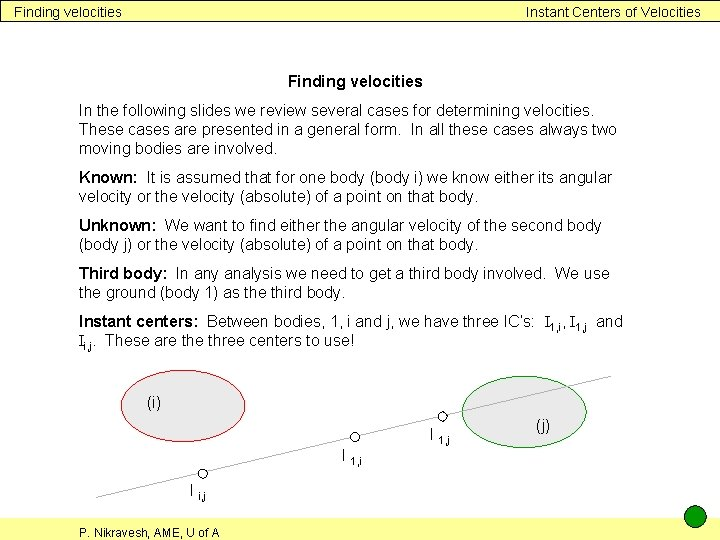Finding velocities Instant Centers of Velocities Finding velocities In the following slides we review several cases for determining velocities. These cases are presented in a general form. In all these cases always two moving bodies are involved. Known: It is assumed that for one body (body i) we know either its angular velocity or the velocity (absolute) of a point on that body. Unknown: We want to find either the angular velocity of the second body (body j) or the velocity (absolute) of a point on that body. Third body: In any analysis we need to get a third body involved. We use the ground (body 1) as the third body. Instant centers: Between bodies, 1, i and j, we have three IC’s: I 1, i, I 1, j and Ii, j. These are three centers to use! (i) I 1, i I i, j P. Nikravesh, AME, U of A I 1, j (j)Problem 1 Instant Centers of Velocities Problem 1 Assume ωi is known and we need to find ωj. Solution: We solve this problem in two steps. ωi (i) I 1, j Ii, j P. Nikravesh, AME, U of A I 1, i (j)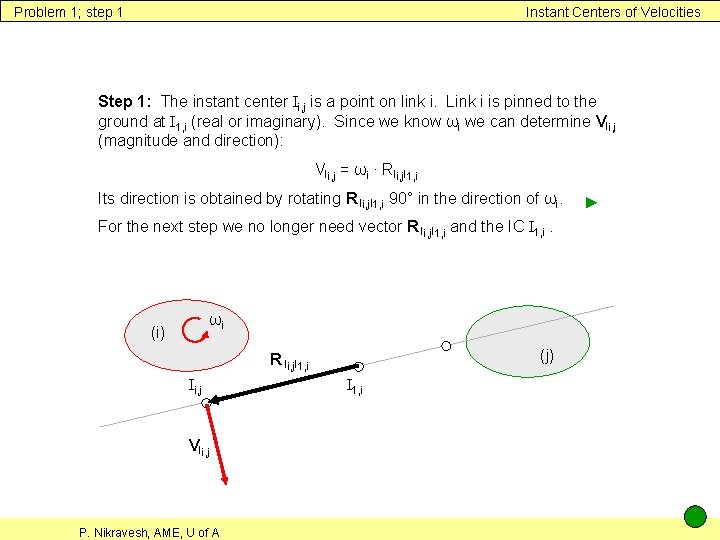Problem 1; step 1 Instant Centers of Velocities Step 1: The instant center Ii, j is a point on link i. Link i is pinned to the ground at I 1, i (real or imaginary). Since we know ωi we can determine VIi, j (magnitude and direction): VIi, j = ωi ∙ RIi, j. I 1, i Its direction is obtained by rotating R Ii, j. I 1, i 90° in the direction of ωi. For the next step we no longer need vector R Ii, j. I 1, i and the IC I 1, i. ωi (i) (j) R Ii, j. I 1, i Ii, j VIi, j P. Nikravesh, AME, U of A I 1, i ►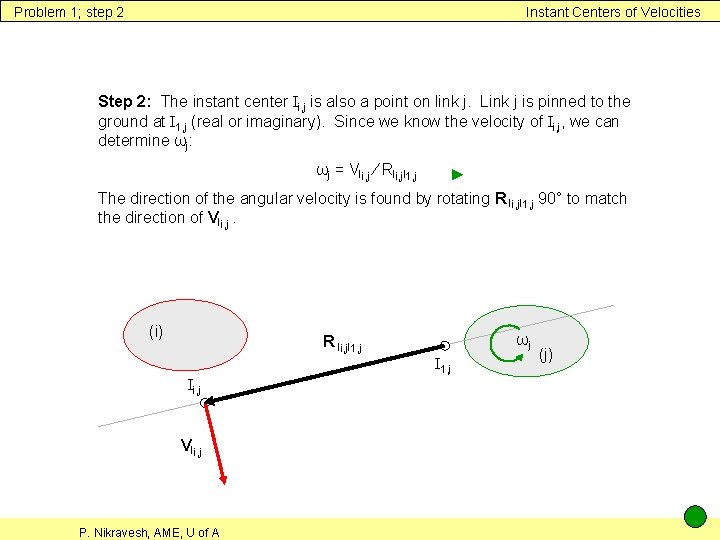Problem 1; step 2 Instant Centers of Velocities Step 2: The instant center Ii, j is also a point on link j. Link j is pinned to the ground at I 1, j (real or imaginary). Since we know the velocity of Ii, j, we can determine ωj: ωj = VIi, j ∕ RIi, j. I 1, j ► The direction of the angular velocity is found by rotating R Ii, j. I 1, j 90° to match the direction of VIi, j. (i) ωj R Ii, j. I 1, j Ii, j VIi, j P. Nikravesh, AME, U of A (j)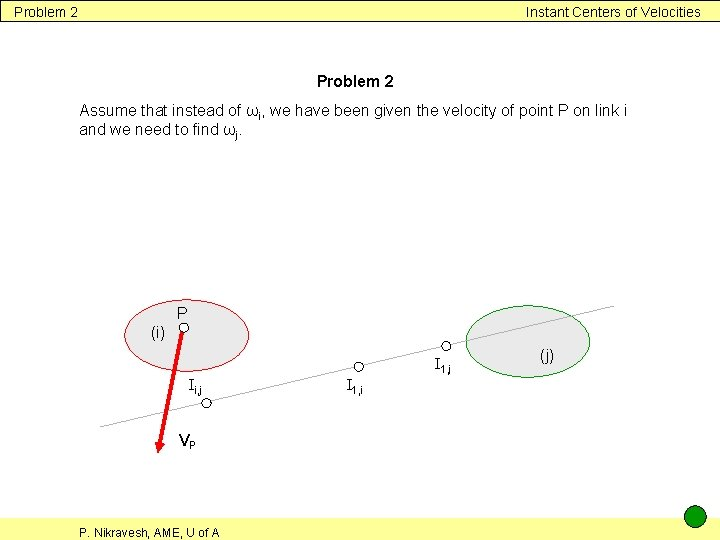Problem 2 Instant Centers of Velocities Problem 2 Assume that instead of ωi, we have been given the velocity of point P on link i and we need to find ωj. P (i) I 1, j Ii, j VP P. Nikravesh, AME, U of A I 1, i (j)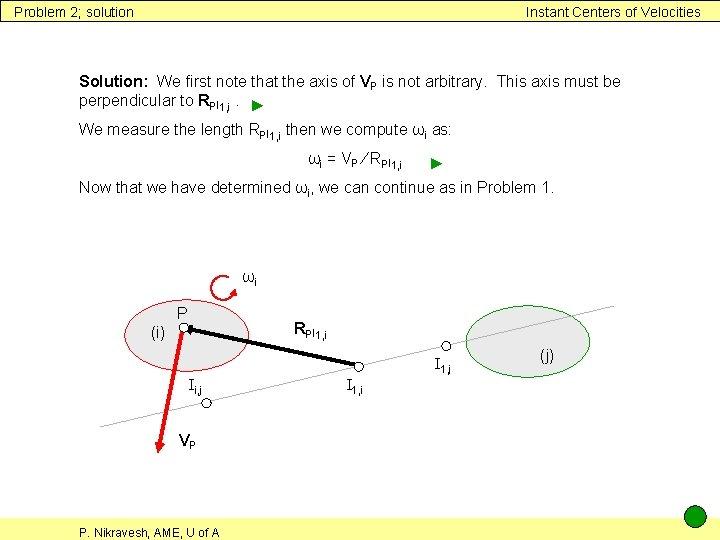Problem 2; solution Instant Centers of Velocities Solution: We first note that the axis of VP is not arbitrary. This axis must be perpendicular to RPI 1, i. ► We measure the length RPI 1, i then we compute ωi as: ωi = VP ∕ RPI 1, i ► Now that we have determined ωi, we can continue as in Problem 1. ωi P RPI 1, i (i) I 1, j Ii, j VP P. Nikravesh, AME, U of A I 1, i (j)Problem 3 Instant Centers of Velocities Problem 3 In this problem we add one more unknown to Problem 1 (or Problem 2). Determine the velocity of point B on link j. ωi (i) B I 1, j Ii, j P. Nikravesh, AME, U of A I 1, i (j)Problem 3; solution Instant Centers of Velocities Solution: We start solving this problem as in Problem 1 (or Problem 2) until we have ωj. ► After that we can easily find the velocity of point B: VB = ωj ∙ RBI 1, j ► ωj ωi (i) B I 1, j Ii, j P. Nikravesh, AME, U of A VB I 1, i RBI 1, j (j)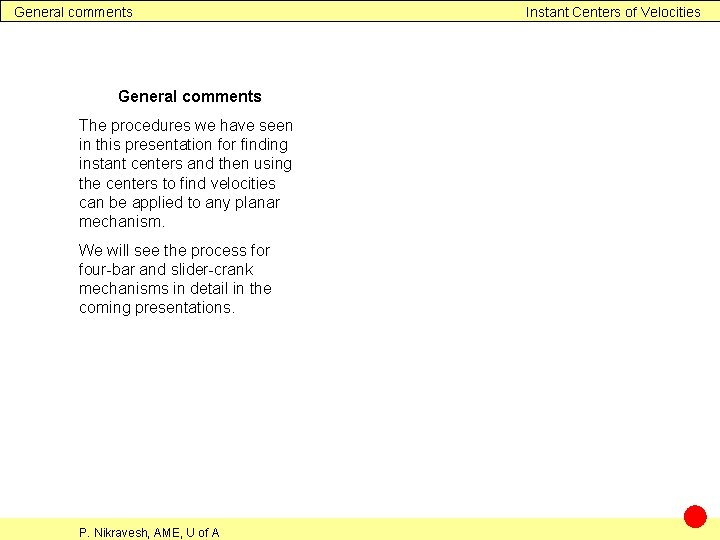General comments The procedures we have seen in this presentation for finding instant centers and then using the centers to find velocities can be applied to any planar mechanism. We will see the process for four-bar and slider-crank mechanisms in detail in the coming presentations. P. Nikravesh, AME, U of A Instant Centers of Velocities